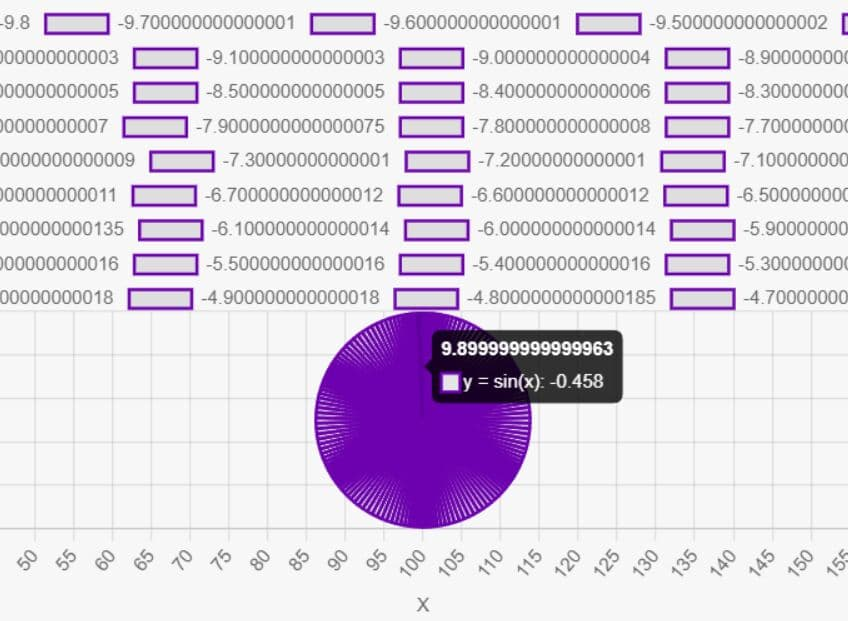# Visualize Mathematical Equations As Graphs - IntrepidGraph

File Size: 6.98 KB 0 11/02/2023 02:11:00 UTC 11/02/2023 02:12:00 UTC Go to website MITIntrepidGraph is a jQuery-powered mathematical graphing calculator to graph various mathematical equations including sin, cos, tan, sqrt, log, exp, pi, e, and more.

After a user inputs an equation, IntrepidGraph calculates y-values for the equation over a range of x-values and then renders the result as an interactive line, bar, pie or scatter chart using Chart.js.

## How to use it:

1. Load the necessary jQuery and Chart.js libraries in the document.

```<!-- Chart.js -->
<script src="https://cdn.jsdelivr.net/npm/chart.js"></script>

<!-- jQuery -->
<script src="/path/to/cdn/jquery.min.js"></script>
```

2. Download the package and load the main script `calculator.js` after jQuery.

```<script src="calculator.js"></script>
```

3. Create the HTML for the IntrepidGraph mathematical graphing calculator.

```<section id="calculator">
<form id="calculatorForm">
<label for="equations">Enter Equations (comma-separated):</label>
<input type="text" id="equations" placeholder="e.g., y = x^2, y = sin(x)" required>
<small>Supported Functions: sin, cos, tan, sqrt, log, exp, pi, e, and more.</small>
<label for="minX">Min X:</label>
<input type="number" id="minX" value="-10">
<label for="maxX">Max X:</label>
<input type="number" class="form-control" id="maxX" value="10">
<label for="graphType">Graph Type:</label>
<select class="form-control" id="graphType">
<option value="line">Line Chart</option>
<option value="scatter">Scatter Plot</option>
<option value="bar">Bar Chart</option>
<option value="pie">Pie Chart</option>
</select>
<button type="submit">Graph It</button>
<button type="reset">Reset Equation</button>
<button type="button">Reset Graph</button>
</form>
<!-- The Graph Will Be Rendered Here -->
<canvas id="graph"></canvas>
</section>
```

This awesome jQuery plugin is developed by intrepidbird. For more Advanced Usages, please check the demo page or visit the official website.